Select Page

# Rotation Guide

for MITRPAK Right Angle Gearboxes

#### MITRPAK Right Angle Gearboxes offer smooth, reliable transfer of motion in 2-Way (single output) or 3-Way (dual output) configurations.

##### Rotation ratios:
• 1:1, output speed is equal to the input speed
• 2:1 (or 1:2), output speed is half (or double) the input speed
##### Rotation styles:

2-Way:

• C1 (output rotates in the same direction as input; see below)
• C2 (output rotates in the reverse direction as input; see below)

3-Way:

• T (output shafts rotate in the same direction; see below)
• C (output shafts rotate in opposite directions; see below)

## 2-Way Right Angle Gearboxes (Single Output)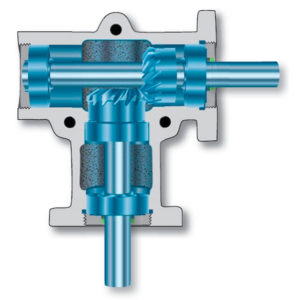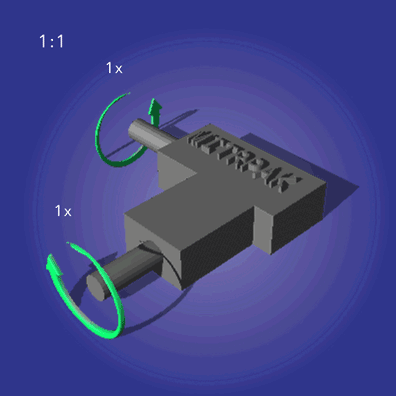Rotation: C1 (same as input)
Ratio: 1:1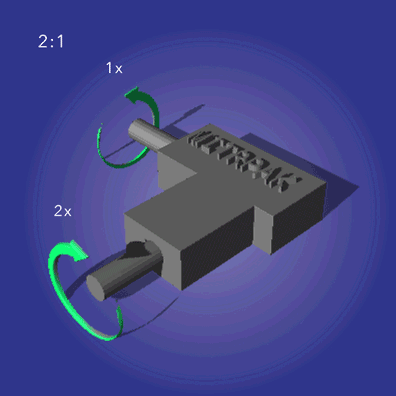Rotation: C1 (same as input)
Ratio: 2:1 or 1:2Rotation: C2 (reverse from input)
Ratio: 1:1Rotation: C2 (reverse from input)
Ratio: 2:1 or 1:2

## 3-Way Right Angle Gearboxes (Dual Output)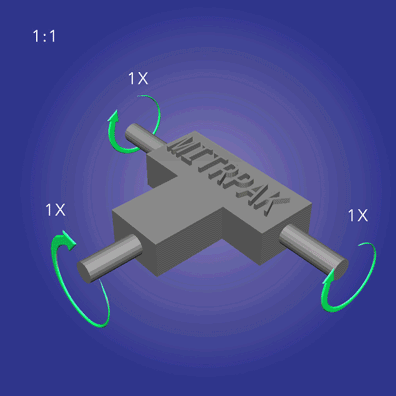Rotation: T (same direction)
Ratio: 1:1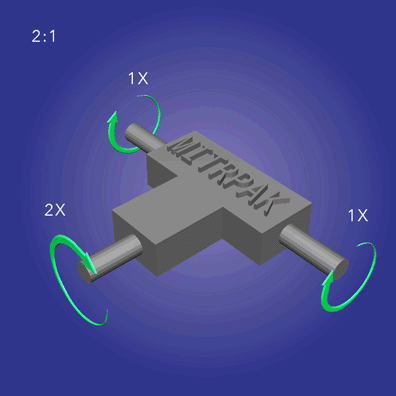Rotation: T (same direction)
Ratio: 2:1 or 1:2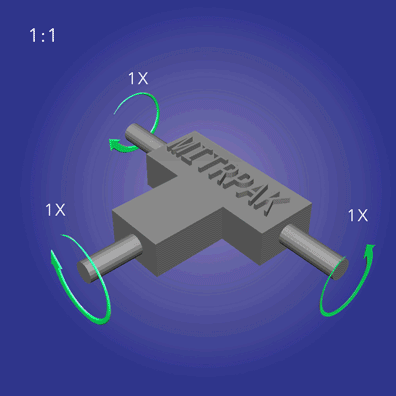Rotation: C (outputs counter-rotate)
Ratio: 1:1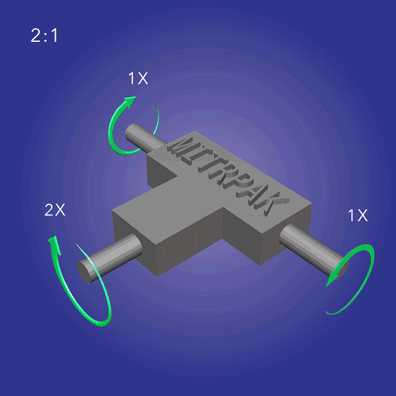Rotation: C (outputs counter-rotate)
Ratio: 2:1 or 1:2